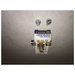Model#### Schneider A .78

See: Square D A .78Square D A .78 Overload Relay Thermal Unit#### Schneider A .95

See: Square D A .95Square D A .95 Overload Relay Thermal Unit#### Schneider A 1.02

See: Square D A 1.02Square D A 1.02 Overload Relay Thermal Unit#### Schneider A 1.25

See: Square D A 1.25Square D A 1.25 Overload Relay Thermal Unit#### Schneider A 1.54

See: Square D A 1.54Square D A 1.54 Overload Relay Thermal Unit#### Schneider A 1.99

See: Square D A 1.99Square D A 2.81 Overload Relay Thermal Unit#### Schneider A 11.9

See: Square D A 11.9Square D A 11.9 Overload Relay Thermal Unit#### Schneider A 13.2

See: Square D A 13.2Square D A 13.2 Overload Relay Thermal Unit#### Schneider A 16.2

See: Square D A 16.2Square D A 16.2 Overload Relay Thermal Unit#### Schneider A 2.57

See: Square D A 2.57Square D A 2.57 Overload Relay Thermal Unit